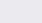Course Content

0/4

0/3

0/1

0/2

0/1

0/4

0/3

0/5

0/3

0/5

0/5

0/1

0/6

0/2

0/4

0/5

(1)

Solve the following triangles, answers of angle correct to 1’, sides correct to 2 decimal places.

(a)  a = 3, b = 6, c = 8

(b)  a = 10, b = 8, C = 30(a)  A = 18.57, B = 39.56, C = 121.87(b)  C = 5.04, B = 52.53, A = 97.47(2)

In triangle ABC, the lengths of the sides AB, BC and CA are 7cm, 2cm and b cm respectively, and the size of angle C is 30. Use the cosine formula to show that, and hence find the exact value of b. Hence, or otherwise, show that(3),, find,.

15, 135(4)Given that BD = 15, AB = 16, AD = 10 andBDC = 30; find the angle of A and the length of BC.

65.83, 8.22

(5)

In

*** QuickLaTeX cannot compile formula:
\bigtriangleupABC

*** Error message:
Undefined control sequence \bigtriangleupABC.
leading text: $\bigtriangleupABC  , the lengths of the sides are three consecutive integers, the greatest angle is twice that of the least, find the lengths of sides of the triangles. *** QuickLaTeX cannot compile formula: \bigtriangleupABC *** Error message: Undefined control sequence \bigtriangleupABC. leading text:$\bigtriangleupABC



ABC ，其边长为三连续整数，最大的角是最小的两倍，求三边长。
（提示：In a triangle,. Show that triangleis a right angle triangle or isosceles triangle.